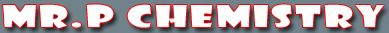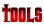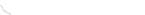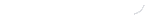activity seriesbond energybond lengthscalculatorcommon namesconversion factors: energyconversion factors: lengthconversion factors: massconversion factors: pressureconversion factors: temp.conversion factors: volumecovalent prefixesdensity solverdiatomic elementse-config. chartelement listgas law formulasKa's of polyprotic acidsKa's of weak acidsKb's of weak basesmetric conversion chartmole conversion chartmolecular geometriesorganic prefixesperiodic tableperiodic table (flash)pH/pOH converterpolyatomic ionspressure converterSI unitssolubility chartsolubility of salts rulessolubility product constantsstoichiometry charttemp. conversiontemp. formulasthermodynamic datavapor pressure of water
SI Units

In 1960 an international agreement was made that established a basic set of metric units for scientific use. These units, known as SI units after the French Systeme Internationale d’Unites are our standard system of measurement units. The SI units we use in chemistry are listed in the chart below.

 International System of Units Quantity SI Unit Length meter (m) Volume cubic meter (m3) Mass kilogram (kg) Density grams per cubic centimeter (g/cm3) grams per milliliter (g/mL) Temperature kelvin (K) Time second (s) Pressure pascal (Pa) Energy joule (J) Amount of a Substance mole (mol)

 You may also find these related tutorials helpfulCompounds in Crust of EarthDensityElements in Crust of EarthHow To Round NumbersMetric ConversionScientific NotationSI UnitsSignificant Figure CalculationsSignificant Figures